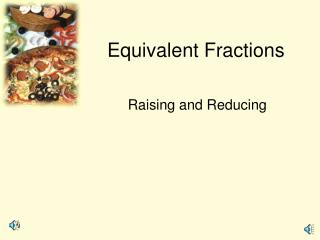DownloadDownload PresentationEquivalent Fractions

# Equivalent Fractions

Télécharger la présentation## Equivalent Fractions

- - - - - - - - - - - - - - - - - - - - - - - - - - - E N D - - - - - - - - - - - - - - - - - - - - - - - - - - -
##### Presentation Transcript

1. Equivalent Fractions Raising and Reducing

2. Pizza

3. Pizza 3/4 9/12 6/8

4. Multiply by 1 • 5 x 1 = • 235 x 1 = • 2/3 x 1 = • a x 1 = 5 235 2/3 a

5. What is 1?

6. Rasing Fractions

7. Rasing Fractions • A man walks into the pizza store and orders a medium pizza. • The clerk asks “ Would you like that cut in 8 pieces or 6?” • The man replied “ Make it 6. I don’t think I can eat 8.”

8. Rasing Fractions • To raise fractions make the denominator larger. • Multiply numerator and denominator (top and bottom) of the fraction by the same number. • This is the same thing as multiplying by 1.

9. Rasing Fractions • Multiply numerator and denominator (top and bottom) of the fraction by the same number. • This is the same thing as multiplying by 1.

10. Rasing Fractions • Raise 3/8 to 56ths. • Multiply numerator and denominator (top and bottom) of the fraction by the same number.

11. Rasing Fractions • Raise 3/8 to 56ths. • Multiply numerator and denominator (top and bottom) of the fraction by the same number.

12. Reducing Fractions • Opposite of raising. • Make the denominator smaller. • Divide numerator and denominator by the same number.

13. Reducing Fractions • Might take more than one step. • More than one way. • Divide numerator and denominator by the same number.

14. Reducing Fractions • Divide numerator and denominator by the same number.

15. Reducing Fractions • Divide numerator and denominator by the same number.

16. Proper and Improper • An improper fraction has a numerator larger than the denominator. • A proper fration has a numerator smaller than the denominator.

17. Mixed vs. Improper • A mixed number has a whole and fraction part. • A mixednumber can be written as an improper and visa versa.

18. Work this on paper. • We have 5 full crates of apples and one crate with only7 apples. The full crates contain 30 apples. • How many apples altogether?

19. Work this on paper. • We have 5 full crates of apples and one crate with only7 apples. The full crates contain 30 apples. • How many apples altogether? • 157 apples. • How did you get the answer?

20. Mixed to Improper. • You multiplied the 5 times 30 and added the 7 • To change a mixed number to improper, multiply the denominator by the whole part and add the numerator. Keep the denominator the same.

21. Mixed to improper • Try some.

22. Mixed to improper • Try some.

23. Try this on paper. • We have 135 pencils and boxes that hold 12 each. • How many full boxes? How many loose pencils? • How did you get your answer?

24. Try this on paper. • We have 135 pencils and boxes that hold 12 each. • How many full boxes? 11 How many loose pencils? 3 • How did you get your answer? • Divided 135 by 12. The remainder was the left over. • 135/12 = 11 3/12

25. Improper to Mixed • Divide.

26. Try these.